<!--查看注释有惊喜-->

## 0x00 前言

### 参考的网站

• https://andrea.corbellini.name/2015/05/17/elliptic-curve-cryptography-a-gentle-introduction
• https://andrea.corbellini.name/2015/05/23/elliptic-curve-cryptography-finite-fields-and-discrete-logarithms/

### 相关代码

• 本文的完整代码
• https://github.com/ninthDevilHAUNSTER/ecc_learning
• Schoof 算法Python版本
• https://github.com/pdinges/python-schoof

### 预先准备的数学概念

#### 群 Group

• 具有封闭性
• 满足结合律
• 存在单位元
• 每个数存在逆元（备注，知乎上的翻译翻错了）
• 满足交换律

#### 模运算 mod

• 模运算的加法
• 模运算的乘法
• 乘法逆元，简称逆元
• 得出 所以，-1是45对模23的逆元
• 关于乘法逆元可以用辗转欧几里得算法来快速求出。代码在misc/shaobaobaoer_math_lab.py下。

#### 形如document.write(katex.renderToString(" y^2 \\;\\equiv \\;A\\;(\\;mod\\;p\\;)"));的同余方程求解问题

https://blog.csdn.net/ACdreamers/article/details/10182281
C代码模板：
https://blog.csdn.net/Quack_quack/article/details/50189111

## 0x01 椭圆曲线定义

### 1.1 标准形式的椭圆曲线绘制

# draw_curve/draw.py
from matplotlib import pyplot as plt
import numpy as np
a = -2
b = 3
y, x = np.ogrid[-5:5:100j, -5:5:100j]
plt.contour(x.ravel(), y.ravel(), pow(y, 2) - pow(x, 3) - x * a - b, )
plt.grid()
plt.legend()
plt.show()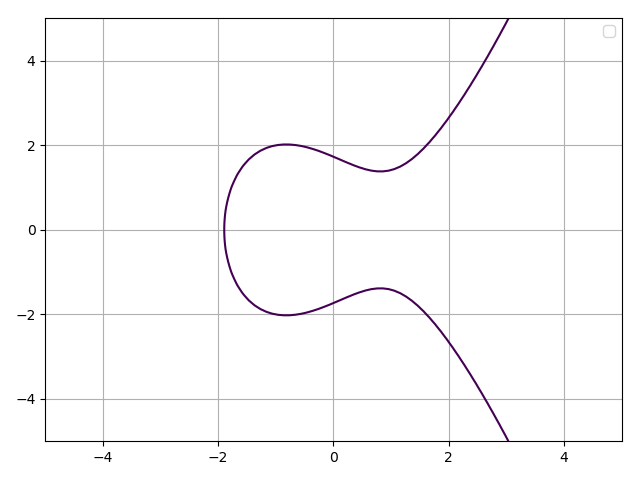### 1.2 椭圆曲线上的群G

• 群众元素满足上头的表达式，也就是说，群中元素是椭圆曲线上的点
• 单位元是无穷远处的点
• 逆元是原坐标点关于x轴对称的那一点。
• 定义一种在该群上的加法，取一条与曲线相交于三点的直线，记交点为，他们的总和等于0，就是说。这三点没有顺序，所以可以证明该椭圆曲线满足交换律。

### 1.3 群G上加法运算的各种情况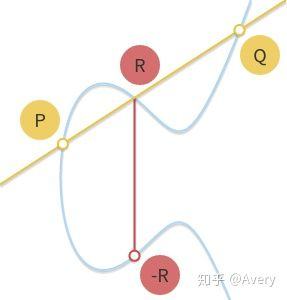#### CASE 3 P != Q 并且 P + Q = -P OR P + Q = -Q:

    def get_three_pionts(self, P, Q):
if P == (0, 0) or Q == (0, 0):
return Q if P == (0, 0) else P

if self.__check_on_curve(P) and self.__check_on_curve(Q):
if P == Q:
# CASE 1
k = (3 * P ** 2 + self.a) / (2 * P)
b = P - k * P
x = k ** 2 - P - Q
y = k * x + b
R = (x, -y)
else:
# CASE 2
k = (P - Q) / (P - Q)
b = P - k * P
x = k ** 2 - P - Q
y = k * x + b
R = (x, -y)
return R
else:
raise ValueError


### 1.4 标量积与对数问题

OK，对于给定的）和是否能够找到这个n呢？当然，这玩意儿不叫除，而是叫对数。（为了和其他密码中“幂”的说法保持一致，从某种程度上而言，这个也算是幂而非数乘）

n = 151, 二进制表示： ，这个二进制也可以表示成幂次加和：

    def get_scalar_multiplication(self, n, P):
"""
Returns the result of n * x, computed using
"""
self.R = (0, 0)
self.P = P
for bit in bits(n):
if bit == 1:
self.R = self.get_three_pionts(self.P, self.R)
self.P = self.get_three_pionts(self.P, self.P)
return self.R


## 0x02 有限域和离散对数领域的椭圆曲线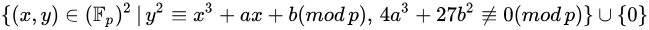a=-7;b=10;p=19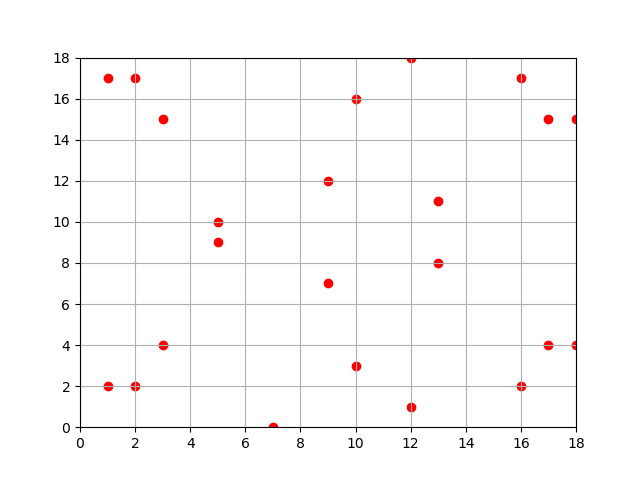a=-7;b=10;p=97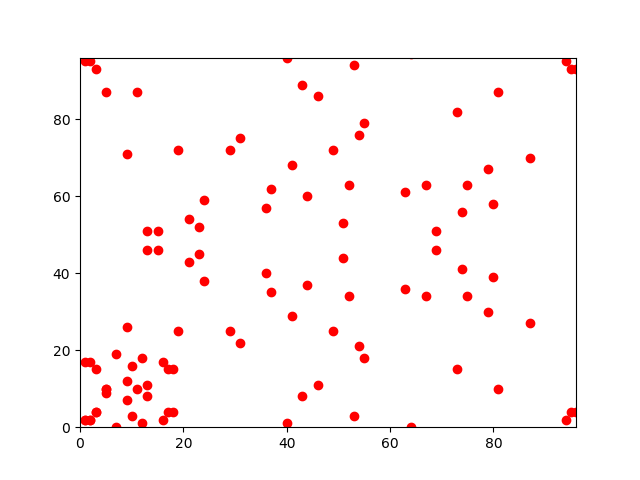### 2.1 离散域上点的加法

#### CASE 3 P != Q 并且 P + Q = -P OR P + Q = -Q:

    def get_three_pionts(self, P, Q):
R = (0, 0)
if P == (0, 0) or Q == (0, 0):
return Q if P == (0, 0) else P
if self.__check_on_curve(P) and self.__check_on_curve(Q):
# CASE 1 P == Q
if P == Q:
k = (3 * P ** 2 + self.a) * egcd(2 * P, self.p)
k %= self.p
b = P - k * P
b %= self.p
x = k ** 2 - P - Q
y = k * x + b
R = (positive_mod(x, self.p), positive_mod(self.p - y, self.p))
else:
# CASE 2 P != Q
k = (P - Q) * egcd(P - Q, self.p)
k %= self.p
b = P - k * P
b %= self.p
x = k ** 2 - P - Q
y = k * x + b
R = (positive_mod(x, self.p), positive_mod(self.p - y, self.p))
return R
else:
raise ValueError


### 2.2 椭圆曲线阶

➜  python-schoof git:(master) ✗ python3  naive_schoof.py 23 4 2
Counting points on y^2 = x^3 + 4x + 2 over GF<23>: 21


### 2.3 从数乘到循环子群

def get_scalar_multiplication(self, n, P):
if n == 0: return 0, 0
self.R = (0, 0)
self.P = P
for bit in bits(n):
if bit == 1:
self.R = self.get_three_pionts(self.P, self.R)
self.P = self.get_three_pionts(self.P, self.P)
return self.R


• 备注：计算斜率的会出现(n * x + p * y) % p != gcd的情况，这也就意味着该点位于无穷远处

### 2.4 循环子群的阶讨论

• 设一个群G的阶为N。任取一个生成自群G的循环子群，设它的阶数是n，生成元为P， n,P 满足的条件是 nP=0 。就好像之前的 5P=0 。（这里的群说的是有限域内的椭圆曲线，只说思路，没加证明）
• P 的阶和椭圆曲线是有联系的，根据拉格朗日定理，子群的阶是父群的阶的因子。换句话说，如果一个椭圆曲线包含 N 个点，它的一个子群包含 n 个点，那么 n 是 N 的因子。

• 用 Schoof 计算 椭圆曲线的阶
• 从小到大遍历 的所有因子
• 找到最小的 使得

### 2.5 寻找子群与离散对数问题

• 计算椭圆曲线的阶
• 选择一个阶为 的子群，n为素数且为的因子。
• 计算辅因子
• 在曲线上选择一个基点 P
• 计算
• 如果G是0，那么重新选择基点否则找到了阶为，生成元为的子群

ECC有趣的地方在于，它的离散问题看上去比其他密码学中的离散问题难多了。这就说明我们可以用更少的位数的整数 k 做到和其他加密算法一样安全级别的加密效果。